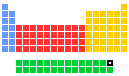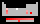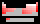▸▸
• 🇬🇧 Ytterbium
• 🇺🇦 Iтербій
• 🇨🇳 鐿
• 🇳🇱 Ytterbium
• 🇫🇷 Ytterbium
• 🇩🇪 Ytterbium
• 🇮🇱 איטרביום
• 🇮🇹 Itterbio
• 🇯🇵 イッテルビウム
• 🇵🇹 Itérbio
• 🇪🇸 Yterbio
• 🇸🇪 Ytterbium
• 🇷🇺 Иттербий

# Ytterbium: radii of atoms and ions70Yb

One measure of size is the element-element distance within the element. It is not always easy to make sensible comparisons between the elements however as some bonds are quite short because of multiple bonding (for instance the O=O distance in O2 is short because of the the double bond connecting the two atoms. The bond length in YbYb is: 388.0pm.

There are several other ways ways to define radius for atoms and ions. Follow the appropriate hyperlinks for literature references and definitions of each type of radius. All values of radii are given in picometres (pm). Conversion factors are:

• 1 pm = 1 × 10‑12 metre (meter)
• 100 pm = 1 Ångstrom
• 1000 pm = 1 nanometre (nm, nanometer)

The size of neutral atoms depends upon the way in which the measurement is made and the environment. Follow the appropriate hyperlinks for definitions of each radius type. The term "atomic radius" is not particularly helpful although its use is widespread. The problem is its meaning, which is clearly very different in different sources and books. Two values are given here, one is based upon calculations and the other upon observation - follow the appropriate link for further details.

The following are calculated values of valence shell orbital radii, Rmax

### References

The Rmax values for neutral gaseous element valence orbitals are abstracted from reference 1.

1. J.B. Mann, Atomic Structure Calculations II. Hartree-Fock wave functions and radial expectation values: hydrogen to lawrencium, LA-3691, Los Alamos Scientific Laboratory, USA, 1968.

This table gives some ionic radii. In this table, geometry refers to the arrangment of the ion's nearest neighbours. Size does depend upon geometry and environment. For electronic configurations, where it matters, the values given for octahedral species are low spin unless stated to be high spin. The terms low spin and high spin refer to the electronic configurations of particular geomtries of certain d-block metal ions. Further information is available in inorganic chemistry textbooks, usually at Level 1 or First Year University level. For definitions of ionic radius and further information, follow the hypertext link.

Yb(II)8-coordinate128Yb(III)8-coordinate112.5Yb(I) -